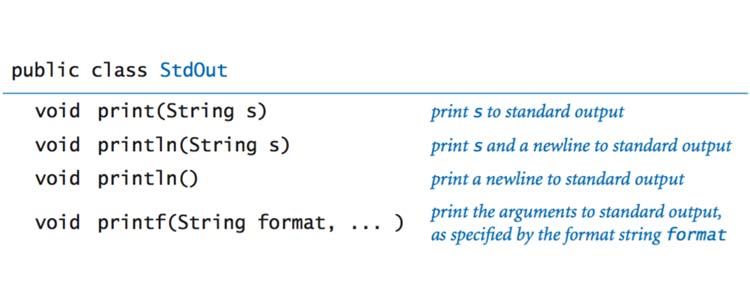# Used to send output to standard output (screen).

System is a class and out is a general static field that takes the output data. Do not worry if you do not notice. We will explain the classes, public and static in the following chapters.

Let’s take an example to print a sentence as output.

1. class AssignmentOperator {
2. public static void main (String [] args) {
3. System.out.println (“Java programming is interesting.”);
4. }
5. }

Output

Java programming is interesting.

Here, println is the method that displays the string inside the quote.

#### What is it? The difference between println () and print (), printf ()

Print () – Prints the string inside the quote.

Println () – Prints the string inside the quote. The cursor then moves to the beginning of the next line.

Printf () – Provides string formatting (similar to printf in C ++ / C programming).

Example 2: print () and println ()

1. class Output {
2. public static void main (String [] args) {
3. System.out.println (“1. println”);
4. System.out.println (“2. println”);
5. System.out.print (“1. print”);
6. System.out.print (“2. print”);
7. }
8. }

Output

1. println

۲. println

1. print 2. print

Do not use quotation marks to display integers, variables, and so on.

Example 3: Printing variables and literals

1. class Variables {
2. public static void main (String [] args) {
3. Double number = -10.6;
4. System.out.println (5);
5. System.out.println (number);
6. }
7. }

Output

5

10.6

You can use the + operator to join strings and print them.

Example 4: Print interconnected strings

1. class PrintVariables {
2. public static void main (String [] args) {
3. Double number = -10.6;
4. System.out.println (“I am” + “awesome.”);
5. System.out.println (“Number =” + number);
6. }
7. }

Output

I am awesome.

Number = -10.6

Consider the following line:

System.out.println (“I am” + “awesome.”);

“I am” and “awesome” strings. Before printing on the page, they are first appended.

In the following line:

System.out.println (“Number =” + number);

First, the value of the number variable is evaluated and converted to a string by the compiler. Then, the strings are collected and printed on the screen.

## Java input

There are several ways to get input from a user in Java. Here you will learn how to use the Scanner object.

To do this, you need to import the Scanner class into the program:

import java.util.Scanner;

Then, we create an object of the Scanner class that is used to receive input from the user.

Scanner input = new Scanner (System.in);

int number = input.nextInt ();

Example 5: Get the input integer from the user

1. import java.util.Scanner;
2. class Input {
3. public static void main (String [] args) {
4. Scanner input = new Scanner (System.in);
5. System.out.print (“Enter an integer:”);
6. int number = input.nextInt ();
7. System.out.println (“You entered” + number);
8. }
9. }

Output

Enter an integer: 23

You entered 23

Here, the input object of the Scanner class is created. The nextInt () method in the Scanner class is then used to receive the integer input from the user.

To get long, float, double and string input from the user, you can use the methods, respectively

nextLong (), nextFloat (), nextDouble () and next ()

use.

Example 6: Get float, double and String inputs

1. import java.util.Scanner;
2. class Input {
3. public static void main (String [] args) {
4. Scanner input = new Scanner (System.in);
5. // Getting float input
6. System.out.print (“Enter float:”);
7. float myFloat = input.nextFloat ();
8. System.out.println (“Float entered =” + myFloat);
9. // Getting double input
10. System.out.print (“Enter double:”);
11. double myDouble = input.nextDouble ();
12. System.out.println (“Double entered =” + myDouble);
13. // Getting String input
14. System.out.print (“Enter text:”);
15. String myString = input.next ();
16. System.out.println (“Text entered =” + myString);
17. }
18. }

Output

Enter float: 2,343

Float entered = 2,343

Enter double: -23.4

Double entered = -23.4

Enter text: Hey!

Text entered = Hey!